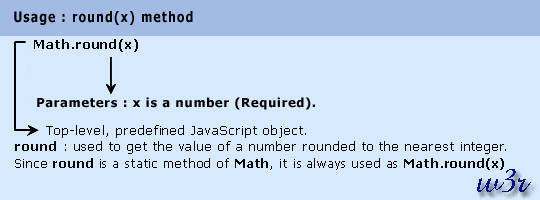# JavaScript round() Method: Math Object

## Description

The round() method of math object is used to get the value of a number rounded to the nearest integer. If the fractional part of the number is greater than or equal to .5, the argument is rounded to the next higher integer. If the fractional part of the number is less than .5, the argument is rounded to the next lower integer.

Version

Implemented in JavaScript 1.0

Syntax

`round(x) `

Parameter

x: Refers a number.Example:

In the following web document, round() method rounded various numbers to their nearest integers.

``````<!DOCTYPE html PUBLIC "-//W3C//DTD XHTML 1.0 Transitional//EN"
"http://www.w3.org/TR/xhtml1/DTD/xhtml1-transitional.dtd">
<html xmlns="http://www.w3.org/1999/xhtml" xml:lang="en" lang="en">
<meta http-equiv="content-type" content="text/html; charset=iso-8859-1" />
<title>JavaScript Math object - round() method example</title>
<body>
<h1 style="color: red">JavaScript Math object : round() method</h1>
<hr />
<script type="text/javascript">
document.write(Math.round(0.99) + "<br />");
document.write(Math.round(0.50) + "<br />");
document.write(Math.round(0.49) + "<br />");
document.write(Math.round(-22.45) + "<br />");
document.write(Math.round(-8.51)+ "<br />");
x=44.50
document.write(Math.round(x));
</script>
</body>
</html>
```
```

View the example in the browser

Supported Browser

 Internet Explorer 7 Firefox 3.6 Google Chrome 7 Safari 5.0.1 Opera 10 Yes Yes Yes Yes Yes